# Mean State

Period Mean (original grids) [Watt m-2]
Model Period Mean (intersection) [Watt m-2]
Model Period Mean (complement) [Watt m-2]
Benchmark Period Mean (intersection) [Watt m-2]
Benchmark Period Mean (complement) [Watt m-2]
Bias [Watt m-2]
RMSE [Watt m-2]
Phase Shift [months]
Bias Score 
RMSE Score 
Seasonal Cycle Score 
Spatial Distribution Score 
Interannual Variability Score 
Overall Score 
Benchmark [-] 179.
CLM5PHSOFF [-] 182. 182. 0.00 177. 216. 5.38 16.6 0.554 0.64 0.52 0.93 0.94 0.73 0.71
CLM5PHSON [-] 183. 183. 0.00 177. 216. 5.83 16.7 0.533 0.63 0.52 0.93 0.94 0.73 0.71
Period Mean (original grids) [Watt m-2]
Model Period Mean (intersection) [Watt m-2]
Model Period Mean (complement) [Watt m-2]
Benchmark Period Mean (intersection) [Watt m-2]
Benchmark Period Mean (complement) [Watt m-2]
Bias [Watt m-2]
RMSE [Watt m-2]
Phase Shift [months]
Bias Score 
RMSE Score 
Seasonal Cycle Score 
Spatial Distribution Score 
Interannual Variability Score 
Overall Score 
Benchmark [-] 189.
CLM5PHSOFF [-] 194. 194. 0.00 188. 224. 5.83 16.4 0.497 0.69 0.53 0.95 1.0 0.78 0.75
CLM5PHSON [-] 195. 195. 0.00 188. 224. 6.89 16.6 0.458 0.66 0.53 0.95 1.0 0.78 0.74
Period Mean (original grids) [Watt m-2]
Model Period Mean (intersection) [Watt m-2]
Model Period Mean (complement) [Watt m-2]
Benchmark Period Mean (intersection) [Watt m-2]
Benchmark Period Mean (complement) [Watt m-2]
Bias [Watt m-2]
RMSE [Watt m-2]
Phase Shift [months]
Bias Score 
RMSE Score 
Seasonal Cycle Score 
Spatial Distribution Score 
Interannual Variability Score 
Overall Score 
Benchmark [-] 194.
CLM5PHSOFF [-] 196. 196. 0.00 192. 221. 3.61 18.9 0.600 0.72 0.56 0.92 0.96 0.67 0.73
CLM5PHSON [-] 197. 197. 0.00 192. 221. 3.85 18.7 0.529 0.72 0.56 0.93 0.96 0.67 0.73
Period Mean (original grids) [Watt m-2]
Model Period Mean (intersection) [Watt m-2]
Model Period Mean (complement) [Watt m-2]
Benchmark Period Mean (intersection) [Watt m-2]
Benchmark Period Mean (complement) [Watt m-2]
Bias [Watt m-2]
RMSE [Watt m-2]
Phase Shift [months]
Bias Score 
RMSE Score 
Seasonal Cycle Score 
Spatial Distribution Score 
Interannual Variability Score 
Overall Score 
Benchmark [-] 81.3
CLM5PHSOFF [-] 37.7 69.3 0.00 80.9 86.2 -11.8 22.4 0.313 0.84 0.74 0.98 0.95 0.69 0.82
CLM5PHSON [-] 72.8 72.9 0.00 80.9 86.2 -9.00 20.1 0.218 0.87 0.75 0.99 0.92 0.70 0.83
Period Mean (original grids) [Watt m-2]
Model Period Mean (intersection) [Watt m-2]
Model Period Mean (complement) [Watt m-2]
Benchmark Period Mean (intersection) [Watt m-2]
Benchmark Period Mean (complement) [Watt m-2]
Bias [Watt m-2]
RMSE [Watt m-2]
Phase Shift [months]
Bias Score 
RMSE Score 
Seasonal Cycle Score 
Spatial Distribution Score 
Interannual Variability Score 
Overall Score 
Benchmark [-] 189.
CLM5PHSOFF [-] 181. 180. 0.00 183. 210. -2.51 20.6 0.661 0.61 0.45 0.91 0.72 0.71 0.64
CLM5PHSON [-] 177. 177. 0.00 183. 210. -2.07 20.5 0.665 0.61 0.46 0.91 0.73 0.71 0.65
Period Mean (original grids) [Watt m-2]
Model Period Mean (intersection) [Watt m-2]
Model Period Mean (complement) [Watt m-2]
Benchmark Period Mean (intersection) [Watt m-2]
Benchmark Period Mean (complement) [Watt m-2]
Bias [Watt m-2]
RMSE [Watt m-2]
Phase Shift [months]
Bias Score 
RMSE Score 
Seasonal Cycle Score 
Spatial Distribution Score 
Interannual Variability Score 
Overall Score 
Benchmark [-] 105.
CLM5PHSOFF [-] 96.1 96.1 0.00 104. 127. -8.15 21.2 0.0810 0.88 0.76 0.99 1.0 0.66 0.84
CLM5PHSON [-] 100. 100. 0.00 104. 127. -3.81 16.7 0.0856 0.93 0.80 0.99 1.0 0.64 0.86
Period Mean (original grids) [Watt m-2]
Model Period Mean (intersection) [Watt m-2]
Model Period Mean (complement) [Watt m-2]
Benchmark Period Mean (intersection) [Watt m-2]
Benchmark Period Mean (complement) [Watt m-2]
Bias [Watt m-2]
RMSE [Watt m-2]
Phase Shift [months]
Bias Score 
RMSE Score 
Seasonal Cycle Score 
Spatial Distribution Score 
Interannual Variability Score 
Overall Score 
Benchmark [-] 140.
CLM5PHSOFF [-] 135. 135. 0.00 139. 154. -4.28 17.3 0.237 0.89 0.74 0.98 1.0 0.63 0.83
CLM5PHSON [-] 136. 135. 0.00 139. 154. -3.72 16.9 0.242 0.89 0.74 0.98 1.0 0.62 0.83
Period Mean (original grids) [Watt m-2]
Model Period Mean (intersection) [Watt m-2]
Model Period Mean (complement) [Watt m-2]
Benchmark Period Mean (intersection) [Watt m-2]
Benchmark Period Mean (complement) [Watt m-2]
Bias [Watt m-2]
RMSE [Watt m-2]
Phase Shift [months]
Bias Score 
RMSE Score 
Seasonal Cycle Score 
Spatial Distribution Score 
Interannual Variability Score 
Overall Score 
Benchmark [-] 180.
CLM5PHSOFF [-] 176. 176. 0.00 178. 210. -2.25 18.9 0.274 0.80 0.62 0.98 0.92 0.63 0.76
CLM5PHSON [-] 175. 175. 0.00 178. 210. -1.80 18.7 0.253 0.81 0.62 0.98 0.92 0.63 0.76
Period Mean (original grids) [Watt m-2]
Model Period Mean (intersection) [Watt m-2]
Model Period Mean (complement) [Watt m-2]
Benchmark Period Mean (intersection) [Watt m-2]
Benchmark Period Mean (complement) [Watt m-2]
Bias [Watt m-2]
RMSE [Watt m-2]
Phase Shift [months]
Bias Score 
RMSE Score 
Seasonal Cycle Score 
Spatial Distribution Score 
Interannual Variability Score 
Overall Score 
Benchmark [-] 74.6
CLM5PHSOFF [-] 61.9 62.3 0.00 74.6 74.8 -12.1 19.6 0.295 0.83 0.74 0.98 0.93 0.67 0.82
CLM5PHSON [-] 64.0 64.4 0.00 74.6 74.8 -11.2 19.0 0.311 0.84 0.74 0.98 0.93 0.67 0.82
Period Mean (original grids) [Watt m-2]
Model Period Mean (intersection) [Watt m-2]
Model Period Mean (complement) [Watt m-2]
Benchmark Period Mean (intersection) [Watt m-2]
Benchmark Period Mean (complement) [Watt m-2]
Bias [Watt m-2]
RMSE [Watt m-2]
Phase Shift [months]
Bias Score 
RMSE Score 
Seasonal Cycle Score 
Spatial Distribution Score 
Interannual Variability Score 
Overall Score 
Benchmark [-] 193.
CLM5PHSOFF [-] 190. 190. 0.00 193. 202. -2.60 18.2 0.219 0.86 0.70 0.98 1.0 0.61 0.81
CLM5PHSON [-] 192. 192. 0.00 193. 202. -1.99 18.1 0.222 0.85 0.70 0.98 1.0 0.61 0.81
Period Mean (original grids) [Watt m-2]
Model Period Mean (intersection) [Watt m-2]
Model Period Mean (complement) [Watt m-2]
Benchmark Period Mean (intersection) [Watt m-2]
Benchmark Period Mean (complement) [Watt m-2]
Bias [Watt m-2]
RMSE [Watt m-2]
Phase Shift [months]
Bias Score 
RMSE Score 
Seasonal Cycle Score 
Spatial Distribution Score 
Interannual Variability Score 
Overall Score 
Benchmark [-] 120.
CLM5PHSOFF [-] 115. 115. 0.00 117. 151. -2.40 16.5 0.350 0.91 0.80 0.98 1.0 0.63 0.85
CLM5PHSON [-] 113. 113. 0.00 117. 151. -1.95 16.4 0.358 0.91 0.80 0.98 1.0 0.62 0.85
Period Mean (original grids) [Watt m-2]
Model Period Mean (intersection) [Watt m-2]
Model Period Mean (complement) [Watt m-2]
Benchmark Period Mean (intersection) [Watt m-2]
Benchmark Period Mean (complement) [Watt m-2]
Bias [Watt m-2]
RMSE [Watt m-2]
Phase Shift [months]
Bias Score 
RMSE Score 
Seasonal Cycle Score 
Spatial Distribution Score 
Interannual Variability Score 
Overall Score 
Benchmark [-] 168.
CLM5PHSOFF [-] 147. 136. 0.00 143. 180. -6.69 19.5 0.289 0.78 0.67 0.97 0.97 0.63 0.78
CLM5PHSON [-] 137. 137. 0.00 143. 180. -5.85 18.9 0.278 0.79 0.67 0.97 0.97 0.62 0.78
Period Mean (original grids) [Watt m-2]
Model Period Mean (intersection) [Watt m-2]
Model Period Mean (complement) [Watt m-2]
Benchmark Period Mean (intersection) [Watt m-2]
Benchmark Period Mean (complement) [Watt m-2]
Bias [Watt m-2]
RMSE [Watt m-2]
Phase Shift [months]
Bias Score 
RMSE Score 
Seasonal Cycle Score 
Spatial Distribution Score 
Interannual Variability Score 
Overall Score 
Benchmark [-] 175.
CLM5PHSOFF [-] 163. 163. 0.00 173. 224. -10.4 19.7 0.309 0.72 0.73 0.97 0.99 0.58 0.79
CLM5PHSON [-] 162. 162. 0.00 173. 224. -10.2 19.7 0.305 0.72 0.73 0.97 0.99 0.58 0.79
Period Mean (original grids) [Watt m-2]
Model Period Mean (intersection) [Watt m-2]
Model Period Mean (complement) [Watt m-2]
Benchmark Period Mean (intersection) [Watt m-2]
Benchmark Period Mean (complement) [Watt m-2]
Bias [Watt m-2]
RMSE [Watt m-2]
Phase Shift [months]
Bias Score 
RMSE Score 
Seasonal Cycle Score 
Spatial Distribution Score 
Interannual Variability Score 
Overall Score 
Benchmark [-] 80.0
CLM5PHSOFF [-] 67.8 67.7 0.00 79.8 82.9 -12.0 20.5 0.0883 0.83 0.76 0.99 0.91 0.73 0.83
CLM5PHSON [-] 70.5 70.4 0.00 79.8 82.9 -10.9 19.6 0.0911 0.84 0.76 0.99 0.89 0.72 0.83
Period Mean (original grids) [Watt m-2]
Model Period Mean (intersection) [Watt m-2]
Model Period Mean (complement) [Watt m-2]
Benchmark Period Mean (intersection) [Watt m-2]
Benchmark Period Mean (complement) [Watt m-2]
Bias [Watt m-2]
RMSE [Watt m-2]
Phase Shift [months]
Bias Score 
RMSE Score 
Seasonal Cycle Score 
Spatial Distribution Score 
Interannual Variability Score 
Overall Score 
Benchmark [-] 205.
CLM5PHSOFF [-] 193. 193. 0.00 200. 227. -6.65 20.4 0.259 0.77 0.64 0.97 0.90 0.63 0.76
CLM5PHSON [-] 192. 192. 0.00 200. 227. -6.23 20.2 0.250 0.77 0.64 0.97 0.89 0.63 0.76
Period Mean (original grids) [Watt m-2]
Model Period Mean (intersection) [Watt m-2]
Model Period Mean (complement) [Watt m-2]
Benchmark Period Mean (intersection) [Watt m-2]
Benchmark Period Mean (complement) [Watt m-2]
Bias [Watt m-2]
RMSE [Watt m-2]
Phase Shift [months]
Bias Score 
RMSE Score 
Seasonal Cycle Score 
Spatial Distribution Score 
Interannual Variability Score 
Overall Score 
Benchmark [-] 153.
CLM5PHSOFF [-] 145. 144. 0.00 151. 194. -6.36 17.0 0.156 0.89 0.76 0.99 1.0 0.63 0.84
CLM5PHSON [-] 144. 144. 0.00 151. 194. -5.31 16.1 0.154 0.91 0.77 0.99 1.0 0.62 0.84
Period Mean (original grids) [Watt m-2]
Model Period Mean (intersection) [Watt m-2]
Model Period Mean (complement) [Watt m-2]
Benchmark Period Mean (intersection) [Watt m-2]
Benchmark Period Mean (complement) [Watt m-2]
Bias [Watt m-2]
RMSE [Watt m-2]
Phase Shift [months]
Bias Score 
RMSE Score 
Seasonal Cycle Score 
Spatial Distribution Score 
Interannual Variability Score 
Overall Score 
Benchmark [-] 115.
CLM5PHSOFF [-] 108. 108. 0.00 114. 123. -6.58 18.2 0.0867 0.89 0.80 0.99 1.0 0.66 0.86
CLM5PHSON [-] 111. 112. 0.00 114. 123. -2.98 14.7 0.0893 0.92 0.83 0.99 1.0 0.64 0.87
Period Mean (original grids) [Watt m-2]
Model Period Mean (intersection) [Watt m-2]
Model Period Mean (complement) [Watt m-2]
Benchmark Period Mean (intersection) [Watt m-2]
Benchmark Period Mean (complement) [Watt m-2]
Bias [Watt m-2]
RMSE [Watt m-2]
Phase Shift [months]
Bias Score 
RMSE Score 
Seasonal Cycle Score 
Spatial Distribution Score 
Interannual Variability Score 
Overall Score 
Benchmark [-] 162.
CLM5PHSOFF [-] 157. 157. 0.00 162. 171. -4.40 15.8 0.166 0.92 0.81 0.99 1.0 0.67 0.86
CLM5PHSON [-] 159. 159. 0.00 162. 171. -2.78 15.1 0.159 0.93 0.81 0.99 1.0 0.65 0.86
Period Mean (original grids) [Watt m-2]
Model Period Mean (intersection) [Watt m-2]
Model Period Mean (complement) [Watt m-2]
Benchmark Period Mean (intersection) [Watt m-2]
Benchmark Period Mean (complement) [Watt m-2]
Bias [Watt m-2]
RMSE [Watt m-2]
Phase Shift [months]
Bias Score 
RMSE Score 
Seasonal Cycle Score 
Spatial Distribution Score 
Interannual Variability Score 
Overall Score 
Benchmark [-] 176.
CLM5PHSOFF [-] 174. 174. 0.00 174. 226. -0.191 17.1 0.499 0.83 0.47 0.94 0.95 0.64 0.71
CLM5PHSON [-] 174. 174. 0.00 174. 226. 0.173 17.1 0.479 0.83 0.47 0.94 0.94 0.64 0.72
Period Mean (original grids) [Watt m-2]
Model Period Mean (intersection) [Watt m-2]
Model Period Mean (complement) [Watt m-2]
Benchmark Period Mean (intersection) [Watt m-2]
Benchmark Period Mean (complement) [Watt m-2]
Bias [Watt m-2]
RMSE [Watt m-2]
Phase Shift [months]
Bias Score 
RMSE Score 
Seasonal Cycle Score 
Spatial Distribution Score 
Interannual Variability Score 
Overall Score 
Benchmark [-] 182.
CLM5PHSOFF [-] 179. 180. 0.00 181. 201. -1.55 19.3 0.356 0.88 0.57 0.96 1.0 0.61 0.76
CLM5PHSON [-] 180. 181. 0.00 181. 201. -0.876 19.1 0.319 0.89 0.57 0.96 1.0 0.61 0.77
Period Mean (original grids) [Watt m-2]
Model Period Mean (intersection) [Watt m-2]
Model Period Mean (complement) [Watt m-2]
Benchmark Period Mean (intersection) [Watt m-2]
Benchmark Period Mean (complement) [Watt m-2]
Bias [Watt m-2]
RMSE [Watt m-2]
Phase Shift [months]
Bias Score 
RMSE Score 
Seasonal Cycle Score 
Spatial Distribution Score 
Interannual Variability Score 
Overall Score 
Benchmark [-] 176.
CLM5PHSOFF [-] 174. 172. 0.00 176. 178. -3.26 20.9 0.321 0.84 0.70 0.97 1.0 0.61 0.80
CLM5PHSON [-] 176. 175. 0.00 176. 178. -3.76 20.8 0.301 0.84 0.70 0.97 1.0 0.60 0.80

# Temporally integrated period mean

BENCHMARK MEAN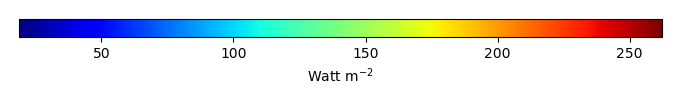MODEL MEANBIAS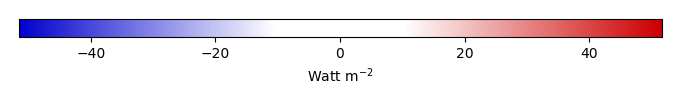BIAS SCORERMSE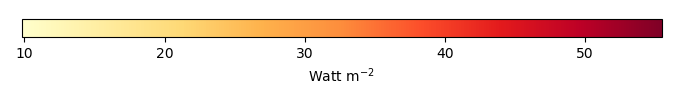RMSE SCOREBENCHMARK INTERANNUAL VARIABILITY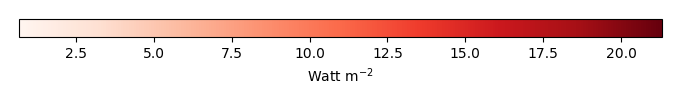MODEL INTERANNUAL VARIABILITYINTERANNUAL VARIABILITY SCOREBENCHMARK MAX MONTHMODEL MAX MONTHDIFFERENCE IN MAX MONTH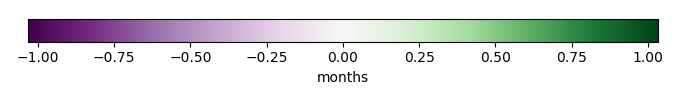SEASONAL CYCLE SCORESPATIAL TAYLOR DIAGRAMMODEL COLORS# Spatially integrated regional mean

MODEL COLORSREGIONAL MEANANNUAL CYCLEMONTHLY ANOMALYANNUAL CYCLE# All Models

BenchmarkCLM5PHSOFFCLM5PHSON# Data Information

creation_date: Tue Jul 1 08:29:23 PDT 2014

source_file: This product is generated from monthly 1 degree GEWEX SRB Radiation observations